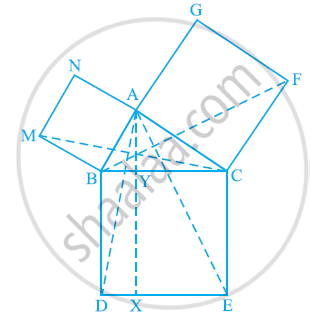# In the following figure, ABC is a right triangle right angled at A. BCED, ACFG and ABMN are squares on the sides BC, CA and AB respectively. Line segment AX ⊥ DE meets BC at Y. Show that - Mathematics

In the following figure, ABC is a right triangle right angled at A. BCED, ACFG and ABMN are squares on the sides BC, CA and AB respectively. Line segment AX ⊥ DE meets BC at Y. Show that:-(i) ΔMBC ≅ ΔABD

(ii) ar (BYXD) = 2 ar(MBC)

(iii) ar (BYXD) = ar(ABMN)

(iv) ΔFCB ≅ ΔACE

(v) ar(CYXE) = 2 ar(FCB)

(vi) ar (CYXE) = ar(ACFG)

(vii) ar (BCED) = ar(ABMN) + ar(ACFG)

Note : Result (vii) is the famous Theorem of Pythagoras. You shall learn a simpler proof of this theorem in Class X.

#### Solution

(i) We know that each angle of a square is 90°.

Hence, ∠ABM = ∠DBC = 90º

⇒ ∠ABM + ∠ABC = ∠DBC + ∠ABC

⇒ ∠MBC = ∠ABD

In ΔMBC and ΔABD,

∠MBC = ∠ABD (Proved above)

MB = AB (Sides of square ABMN)

BC = BD (Sides of square BCED)

∴ ΔMBC ≅ ΔABD (SAS congruence rule)

(ii) We have

ΔMBC ≅ ΔABD

⇒ ar (ΔMBC) = ar (ΔABD) ... (1)

It is given that AX ⊥ DE and BD ⊥ DE (Adjacent sides of square

BDEC)

⇒ BD || AX (Two lines perpendicular to same line are parallel to each other)

ΔABD and parallelogram BYXD are on the same base BD and between the same parallels BD and AX.

∴ ar(ΔABD) = 1/2ar(BYXD)

ar(BYXD) = 2ar(ΔABD)

Area (BYXD) = 2 area (ΔMBC) [Using equation (1)] ... (2)

(iii) ΔMBC and parallelogram ABMN are lying on the same base MB and between same parallels MB and NC.

∴ ar(ΔMBC) = 1/2ar(ABMN)

2ar (ΔMBC) = ar (ABMN)

ar (BYXD) = ar (ABMN) [Using equation (2)] ... (3)

(iv) We know that each angle of a square is 90°.

∴ ∠FCA = ∠BCE = 90º

⇒ ∠FCA + ∠ACB = ∠BCE + ∠ACB

⇒ ∠FCB = ∠ACE

In ΔFCB and ΔACE,

∠FCB = ∠ACE

FC = AC (Sides of square ACFG)

CB = CE (Sides of square BCED)

ΔFCB ≅ ΔACE (SAS congruence rule)

(v) It is given that AX ⊥ DE and CE ⊥ DE (Adjacent sides of square BDEC)

Hence, CE || AX (Two lines perpendicular to the same line are parallel to each other)

Consider ΔACE and parallelogram CYXE

ΔACE and parallelogram CYXE are on the same base CE and between the same parallels CE and AX.

∴ ar(ΔACE) = 1/2ar(CYXE)

⇒ ar(CYXE) = 2 ar(ΔACE) ... (4)

∴ ΔFCB ≅ ΔACE

ar (ΔFCB) ≅ ar (ΔACE) ... (5)

On comparing equations (4) and (5), we obtain

ar (CYXE) = 2 ar (ΔFCB) ... (6)

(vi) Consider ΔFCB and parallelogram ACFG

ΔFCB and parallelogram ACFG are lying on the same base CF and between the same parallels CF and BG.

∴ ar(ΔFCB) = 1/2 ar(ACFG)

⇒ ar(ACFG) = 2 ar(ΔFCB)

⇒ ar (ACFG) = ar (CYXE) [Using equation (6)] ... (7)

(vii) From the figure, it is evident that

ar (BCED) = ar (BYXD) + ar (CYXE)

⇒ ar (BCED) = ar (ABMN) + ar (ACFG) [Using equations (3) and (7)]

Concept: Corollary: Triangles on the same base and between the same parallels are equal in area.
Is there an error in this question or solution?
Chapter 9: Areas of Parallelograms and Triangles - Exercise 9.4 [Page 166]

#### APPEARS IN

NCERT Class 9 Maths
Chapter 9 Areas of Parallelograms and Triangles
Exercise 9.4 | Q 8 | Page 166
RD Sharma Mathematics for Class 9
Chapter 14 Areas of Parallelograms and Triangles
Exercise 14.3 | Q 30 | Page 48

Share